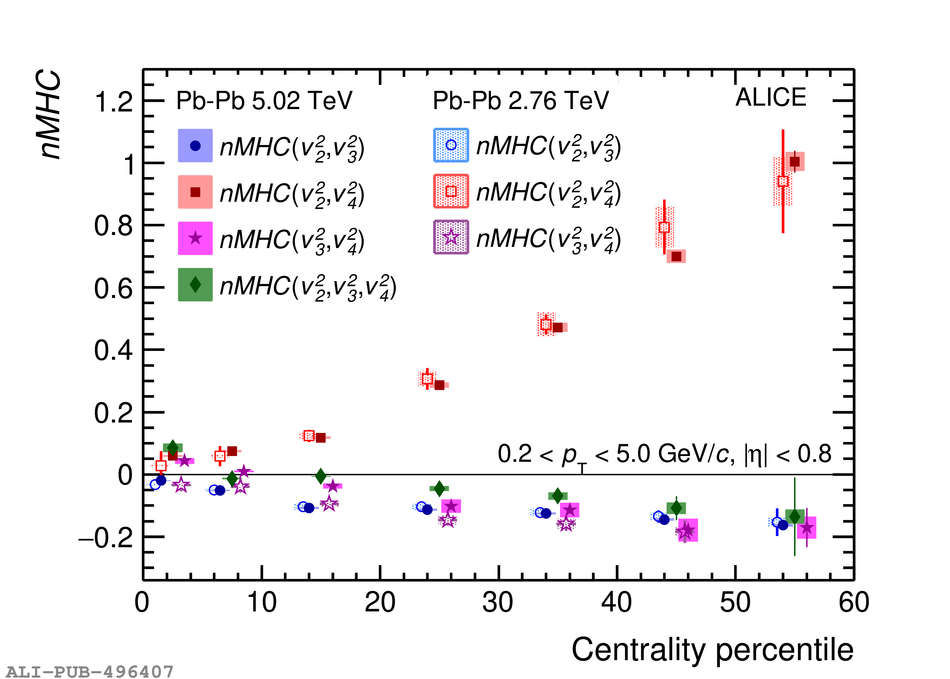# Figure 1

 Centrality dependence of $nMHC(v_{2}^{2}, v_{3}^{2})$, $nMHC(v_{2}^{2}, v_{4}^{2})$, $nMHC(v_{3}^{2}, v_{4}^{2})$ and $nMHC(v_{2}^{2}, v_{3}^{2}, v_{4}^{2})$ in Pb--Pb collisions at $\sqrt{s_{\rm NN}} = 5.02$ TeV, shown by the solid markers. The statistical (systematic) errors are shown with vertical bars (filled boxes). Comparisons to the previous measurements at 2.76 TeV from Refs. , shown by the open markers, are also presented. Data points are shifted for visibility.